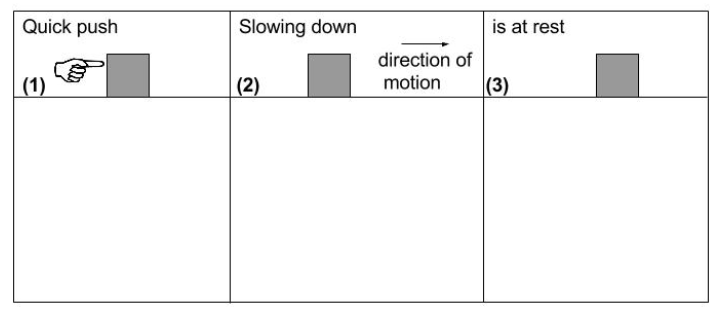Question
6 views

Consider the following 3 pieces of the motion for a block that is initially at rest on a floor. (1) The block's velocity is zero at the instant it is given a quick push by a hand. (2) The hand does not touch the block after the quick push. The block slides across the floor, gradually slows down, then comes to rest. In (3), the block is at rest. The next series of questions will pertain to these cases.

In (1), while the hand is pushing the block, but while the block is still has zero velocity, what is the direction of the block’s acceleration?help_outlineImage Transcriptioncloseis at rest Quick push Slowing down direction of motion |(3) |(1) (2) fullscreen
check_circle

While the hand is pushing the block and the block is still at rest, the acceleration of block is in forward direction i.e. in the direction of the force.

Let's understand this with an example:

When a body is thrown upward, it goes upto a maximum height. At maximum height the velocity of the body becomes zero. While the velcoity is still zero, the gravitational force of earth or force due to gravity does not stop acting in downward direction.  So, at that moment, the acceleration of the body is in downward direction but the velocity of the body is zero.

Similarly, in the given case, When the hand is pushing the box it is applying some force on the box. Due to that force, the box has an acceleration a = F/m in the direction of the force applied. But as the block is at rest initially, so at that moment the velocity of the box is zero.

But as the block has a acceleration due to applied force, the very next moment the velocity of the block will st...

### Want to see the full answer?

See Solution

#### Want to see this answer and more?

Solutions are written by subject experts who are available 24/7. Questions are typically answered within 1 hour.*

See Solution
*Response times may vary by subject and question.
Tagged in
SciencePhysics

### Kinematics